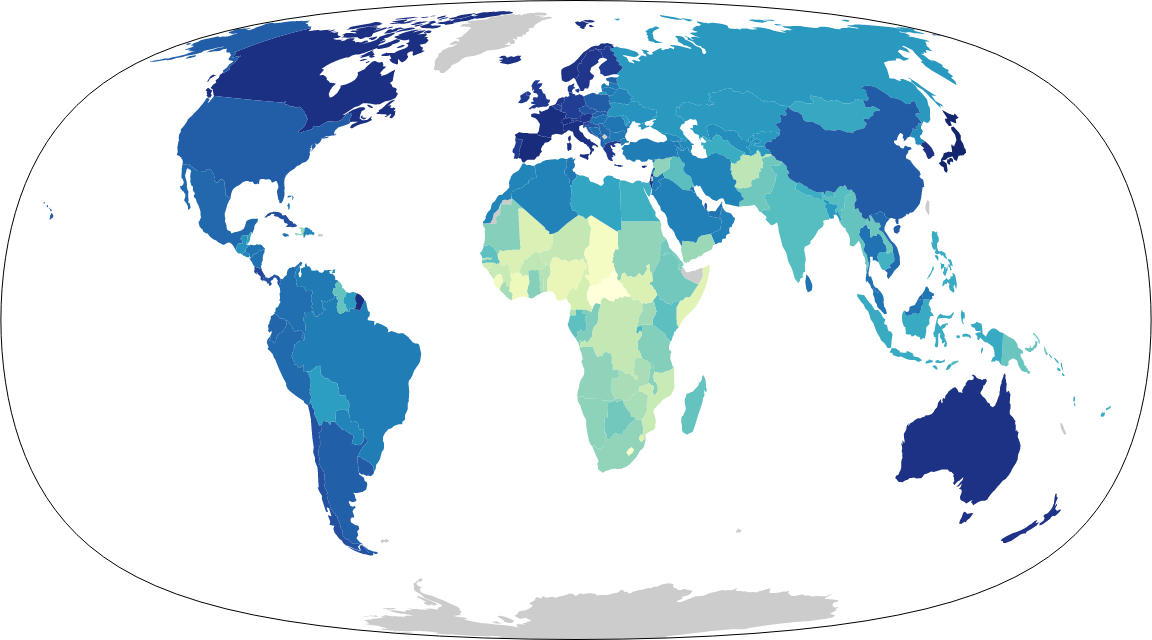# The Sledgehammer projectionThis post relies on some spiffy new browser features, and might not work on your machine. Apologies.

The Sledgehammer projection — named after the Peter Gabriel song — is a novel equal-area map projection designed to fill the same niche as the Winkel Tripel. A composite of the Hammer and Peters projections, it preserves area, gives both parallels and meridians pleasing curves, and with its pointed poles, it does not distort areas in far northern latitudes to the extent that flat-topped projections such as Equal Earth do. (I dare even say that it handles Antarctica alright.)

The exact formula, based on Strebe (2017)’s technique:1

• $\text{Sledge}\left(\lambda ,\phi \right)=\text{p}\left(\text{h}\prime \left[\sqrt{\frac{1}{2}}\cdot \text{h}\left(\lambda ,\phi \right)\right]\right)$, where
• $\text{h}\left(\lambda ,\phi \right)$ refers to the Hammer projection2,
• $\text{h}\prime \left(x,y\right)$ refers to its inverse3, and
• $\text{p}\left(\lambda ,\phi \right)=\left(\frac{8}{9}\cdot \frac{3}{\pi }\lambda ,\sqrt{\frac{4}{3}}\mathrm{sin}\phi \right)$

To invert:

$\text{Sledge}\prime \left(x,y\right)=\text{h}\prime \left(\sqrt{2}\cdot \text{h}\left[\frac{9}{8}\cdot \frac{\pi }{3}x,\mathrm{asin}\left(\sqrt{\frac{3}{4}}y\right)\right]\right)$

And, finally, an equation describing the outer boundary of the map:

${\mathrm{tan}}^{2}\left(\frac{3}{16}\pi x\right)=3-3{y}^{2}$

An interactive version is available here. Happy mapping!

Please be nice. Comments may be edited for proper spelling and capitalisation, because i’m a pedant. Basic formatting: `*bold*`, `/italics/`, `[//satyrs.eu links]`→ More